# Dimensions - Definition with Examples

The Complete K-5 Math Learning Program Built for Your Child

• 40 Million Kids

Loved by kids and parent worldwide

• 50,000 Schools

Trusted by teachers across schools

• Comprehensive Curriculum

Aligned to Common Core

## What are Dimensions?

Dimensions in mathematics are the measure of the size or distance of an object or region or space in one direction. In simpler terms, it is the measurement of the length, width, and height of anything.

Any object or surroundings or space can be

One-dimensional (or 1D)

Two-dimensional ( or 2D)

Three-dimensional (or 3D)

For example,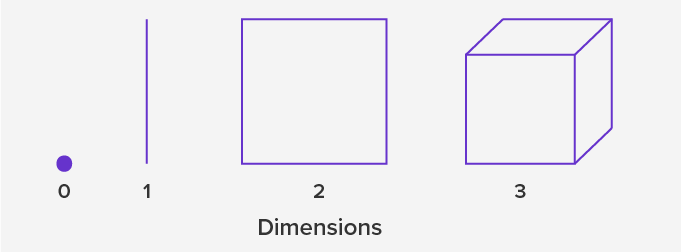## Zero Dimensional

A point is a zero-dimensional object as it has no length, width or height.  It has no size. It tells about the location only.

## One Dimensional

A line segment drawn on a surface is a one-dimensional object, as it has only length and no width.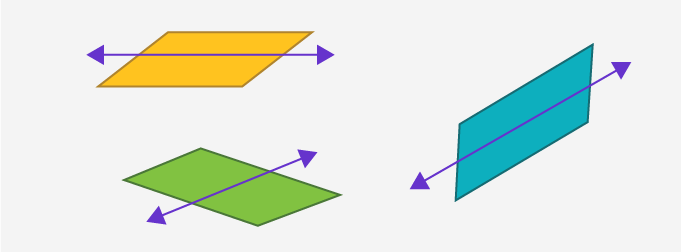## Two Dimensional

The 2-dimensional shapes or objects in geometry are flat plane figures that have two dimensions – length and width.  Two-dimensional or 2-D shapes do not have any thickness and can be measured in only two faces.

A square, circle, rectangle, and triangle are examples of two-dimensional objects.  We can classify figures on the basis of the dimensions they have.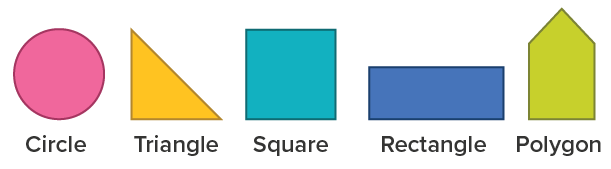## Three dimensional

In geometry, three-dimensional shapes are solid figures or objects or shapes that have three dimensions – length, width, and height. Unlike two-dimensional shapes, three-dimensional shapes have thickness or depth.

A cube and cuboid are examples of three-dimensional objects, as they have length, width, and height.

Take for example a cuboid,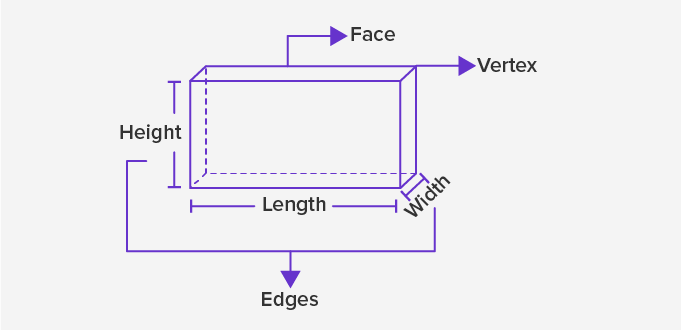The attributes of the cuboid are faces, edges, and vertices. The three dimensions compose the edges of a 3D geometric shape.

Some examples of three-dimensional shapes:

 3-D shapes Examples CubeRubric cube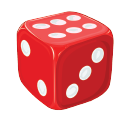Dice SphereBallRound ConeCarrotcone Rectangular prism and cuboidBookGift

Every three-dimensional figure houses many two-dimensional figures. We can by joining together many two-dimensional figures we get a three-dimensional figure.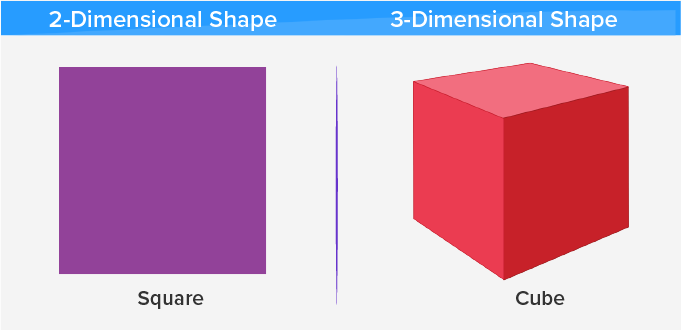Fun fact We can have more than three dimensions in mathematics, but it will be hard to draw on paper.

Let’s do

Identify two-dimensional shapes within the three-dimensional shapes given below.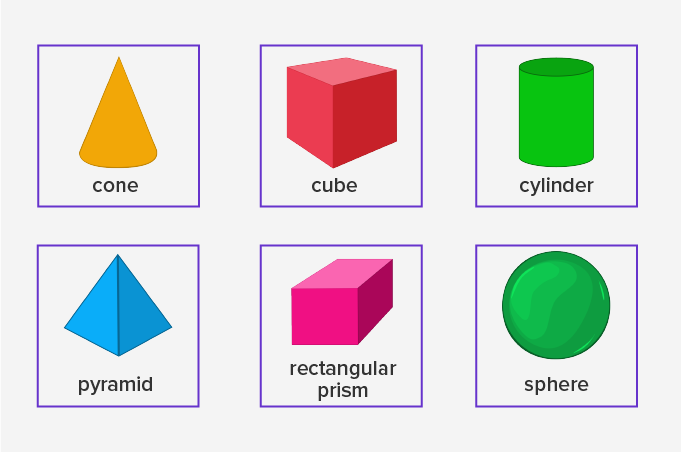Won Numerous Awards & Honors